# Lenses

## SPECIFIC OBJECTIVES

To understand the difference between converging and diverging lenses; to build a working telescope.

## EQUIPMENT

Lenses, lens carriers, optical bench, light source, screen.

## BACKGROUND

Lenses work by bending rays of light in a phenomenon called refraction, the same process we studied in the prism experiment. Refraction occurs whenever light passes from a medium with one index of refraction to another medium with a different index of refraction. When light enters a less optically dense medium (lower index of refraction), it bends away from the perpendicular. When light enters a more optically dense medium (higher index of refraction), it bends toward from the perpendicular.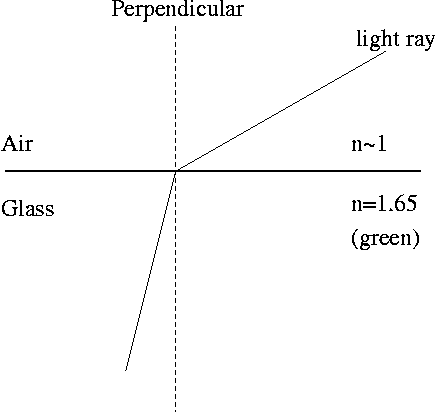The surface of a lens is curved, but the law of refraction stated above still holds at every point on the curved surface.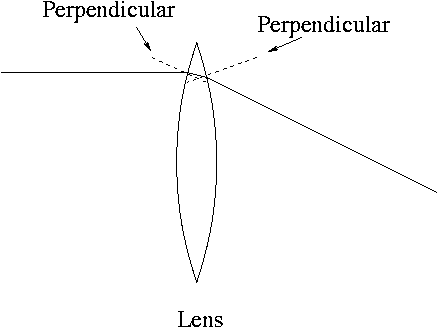The figure below shows the path of some light rays from a point object through the a positive lens. You see that the lens refracts the rays passing through the edge of the lens while not refracting the ray that passes through the center of the lens. All of the rays from a point object converge at the same spot, producing a point image.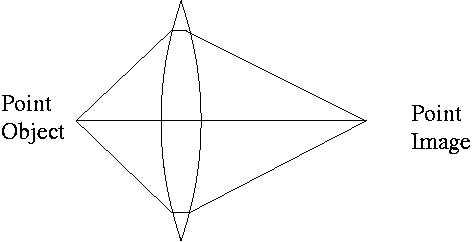When the rays coming from the object are parallel, as occurs for very distant objects (farther away than about 50 focal lengths), an image forms at a distance from the lens called the focal length.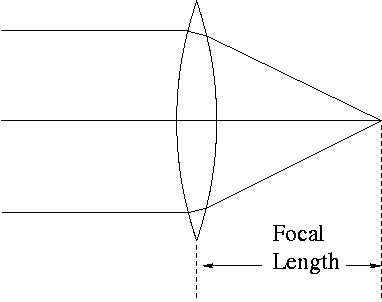The focal length of a lens is determined by the index of refraction of the glass used and by the curvature of its surface. Once the lens is manufactured, its focal length is fixed.

In the figure of a positive eyepiece telescope below, F is the focal length of the objective lens and f is the focal length of the eye lens. The telescope has a theoretical magnifying power given by

M    =    - F / f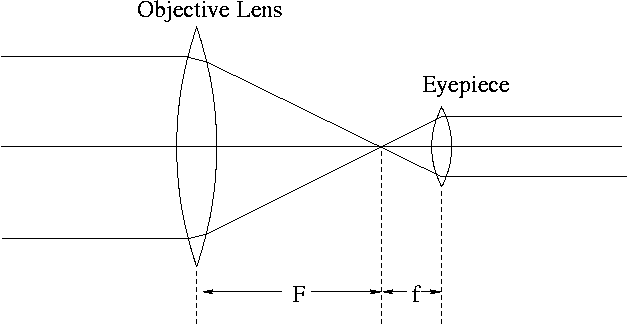There are two kinds of images formed by lenses, real and virtual. A real image can be projected onto a screen. A virtual image appears between the lens and the object and cannot be projected on a screen.

Virtual images can be formed by both converging and diverging lenses. Real images, however, can only be formed by converging lenses.

Try this link to a java lens simulator

## PROCEDURE

### Positive and Negative Lenses

1. Place each of the seven lenses on a page of text. When lifted slightly off the page, some lenses will magnify the text - these are converging or positive lenses. Others will make the text look smaller - these are diverging or negative lenses.

2. Make a table of lens number and lens type.

3. Hold one of the positive lenses at arm's length and look through it at some distant object. Does the image appear to hover between your eye and the lens? Or does the image appear to hover between the lens and the distant object? Is the image upright or inverted? What kind of image is this (real or virtual)?
4. Hold one of the negative lenses at arm's length and look through it at some distant object. Does the image appear to hover between your eye and the lens? Or does the image appear to hover between the lens and the distant object? Is the image upright or inverted? What kind of image is this (real or virtual)?

### Determination of Focal Length

1. Determine the focal length of each positive lens by placing it in the lens carrier between the light source and the screen.

2. Move the light source to one extreme on the optical bench. Move the screen to the other extreme on the bench. The vertical lines on the carriers give the position to the nearest half millimeter.

3. Slide the lens carrier along the optical bench until a sharp image of the source is formed on the screen.

4. Measure the object distance do between the light source and the lens, with an error estimate (the lens can be moved a certain distance with no apparent effect on the sharpness of the image). Measure the image distance di between the lens and the screen, with an error estimate.

5. Find the focal length of each positive lens using the lens grinder's equation:

1 / f    =    1 / do    +    1 / di

6. Is the image on the screen inverted or upright?

7. Is the image on the screen real or virtual?

8. Cover half of the lens with a piece of paper. What happens to the image?

9. Verify that the negative lenses will not form an image on the screen. Therefore, this method cannot be used to determine the focal length of a negative lens.

### Making a Telescope

1. Select the positive lens having the longest MEASURED focal length -- this will be the objective lens. Select another positive lens having the shortest focal length -- this will be the eye lens.

2. Remove the light source and screen from their holders. Mount the objective and eye lenses in carriers and adjust their separation to the sum of their two focal lengths. Position the optical bench so that it is aimed at something far away from you.

3. Put a finger on the small rod that protrudes from the lens carrier to protect your eye and place your eye up close to the eye lens. Look toward the distant object through the eye lens and adjust the separation until you see a clear image. You now have a simple telescope.

4. Compare the apparent size of the image seen through the telescope to the apparent size of the of the object seen with an unaided eye. Record an estimate for the experimental magnification power of the telescope.

## The following Shockwave demonstration was submitted by Jeff Schmidt:

5. Compare the experimental magnification with the theoretical magnification.

6. Is the image viewed through the telescope inverted or upright?

7. Remove the positive eye lens from the carrier and replace it with one of the negative lenses. You will need to move the negative eye lens closer (Why?) to the objective lens to get a clear image of the distant object. Record the separation between the two lenses. This is the type of telescope made by Galileo.

8. Compare the apparent size of the image seen through the telescope to the apparent size of the of the object seen with an unaided eye. Record an estimate for the experimental magnification power of the telescope.

9. Is the image viewed through the telescope inverted or upright?

10. Sketch a ray diagram for this negative eyepiece telescope similar to the figure above for the positive eyepiece telescope. Make your sketch large and clear, noting any significant differences from the diagram of the positive eyepiece telescope.

11. What is the focal length of the negative lens? There are two ways to estimate this -- try them both and compare the results.

## Analysis

1. For each positive lens, find the error in the focal length using

(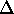f) / f2    =    (do) / do2    +    (di) / di2

2. For the positive eyepiece telescope, find the error in the theoretical magnification. Does your experimental value fall within this error range?
3. Discuss random and systematic errors in this experiment.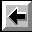Back to the Electricity and Magnetism Manual